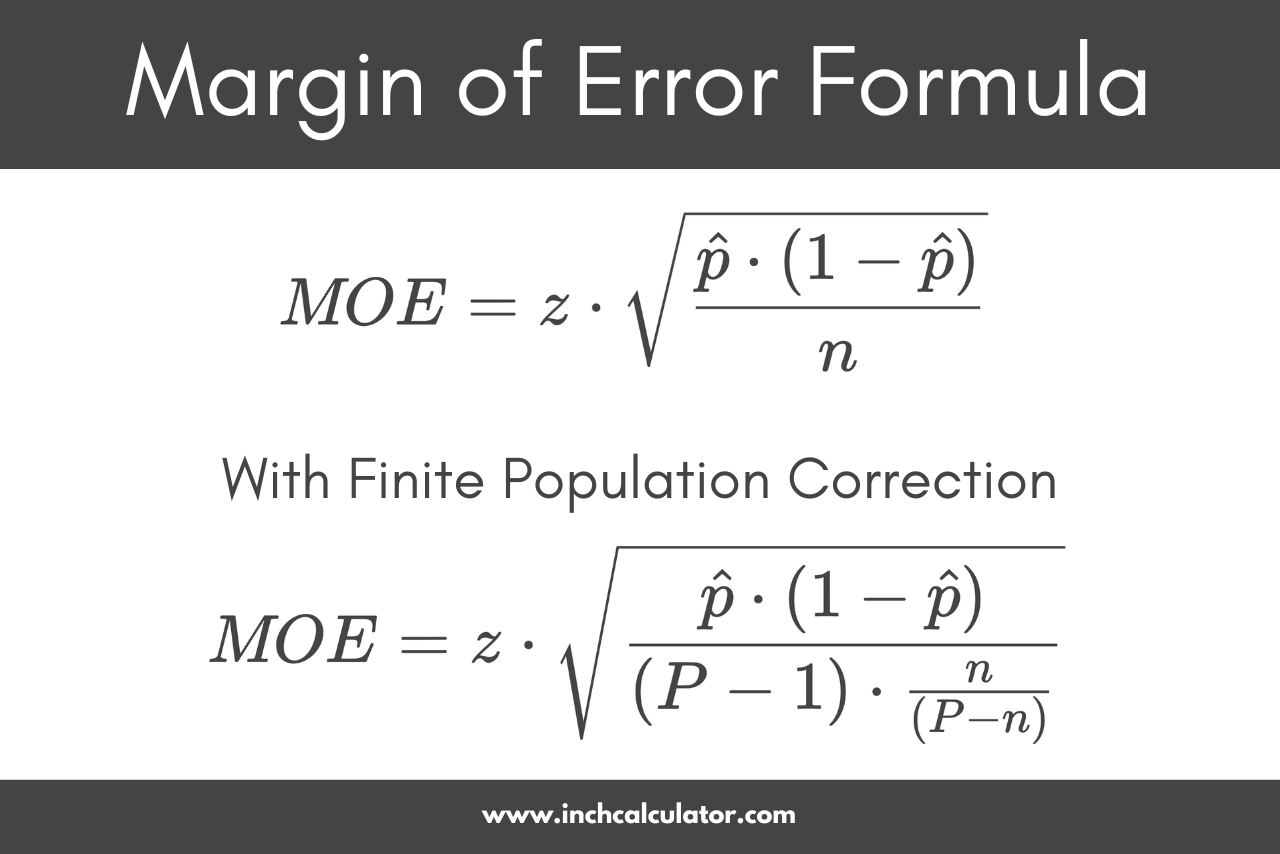# Margin of Error Calculator

Enter the confidence level, sample size, sample proportion, and population size to find the margin of error using the calculator below.

Proportion of the sample with the characteristic of interest
%
optional

## Margin of Error:

### Steps to Solve

#### Margin of Error Formula

MOE = z\cdot\sqrt{\frac{\hat{p} \cdot (1-\hat{p})}{n}}

z-score = ?

#### Step Two: Find the Margin of Error

MOE = ?

Learn how we calculated this below

## How to Find the Margin of Error

In statistics, the margin of error is the amount of error in random sampling surveys. Because surveys are often done on a sample of a larger population, there will inevitably be some amount of error.

A lower margin of error produces higher confidence levels in a survey sample. Thus, the margin of error is a good indicator of the confidence you can have in the sample or survey results.

The margin of error is expressed as a percentage indicating the degree that the sample results differ from the total population, for instance, when calculating a confidence interval.

### Margin of Error Formula

The margin of error is equal to the z-score for the confidence level times the standard error. You can find the margin of error using the following formula:

MOE = z\frac{\sigma}{\sqrt{n}}

Where:
z = critical value for the confidence level (z-score)
σ = standard deviation
n = sample size

Thus, for a normal distribution of data, the margin of error is equal to the z-score z times the population standard deviation σ divided by the square root of the sample size n. You can find the z-score for your desired confidence level in the table below.

#### Margin of Error Formula for a Sample

While you can calculate the standard deviation using a calculator, you can also use the sample proportion to calculate it. The sample proportion is denoted and represents the percentage of the sample that has the characteristic you’re interested in.

The standard deviation σ can be expressed with the formula, where is the sample proportion:

\sigma = \sqrt{\hat{p} \cdot (1-\hat{p})}

By substituting this formula for the standard deviation into the formula above, you can calculate the margin of error using this formula:

MOE = z\cdot\sqrt{\frac{\hat{p} \cdot (1-\hat{p})}{n}}

Where:
z = critical value for the confidence level (z-score)
= sample proportion
n = sample size

Thus, the margin of error is equal to the z-score z times the standard error, which is the square root of the sample proportion times 1 minus the sample proportion , divided by the sample size n.

#### Margin of Error Formula with Finite Population Correction

To calculate the margin of error with finite population correction, you can use the following formula:

MOE = z\cdot\sqrt{\frac{\hat{p} \cdot (1-\hat{p})}{(P-1)\cdot\frac{n}{(P-n)}}}

Where:
z = critical value for the confidence level (z-score)
= sample proportion
n = sample size
P = population size

The margin of error with finite population correction is equal to the z-score z times the square root of the sample proportion times 1 minus the sample proportion , divided by the population size P minus 1, times the sample size n divided by the population size P minus the sample size n.### Example

Let’s find the margin of error for a survey asking people what their favorite color is. The survey sampled 250 people, and 40% of respondents answered that their favorite color was red. We want a 95% confidence level in the margin of error.

Start by assigning values to the variables needed for the formula.

z = 1.96 (95% confidence = 1.96 [table])
= 0.4 40% ÷ 100 = 0.4
n = 250 sample size

Next, substitute the variables in the formula and solve.

1.96\cdot\sqrt{\frac{0.4 \cdot (1-0.4)}{250}}
1.96\cdot\sqrt{\frac{0.4 \cdot 0.6}{250}}
1.96\cdot\sqrt{0.00096}
1.96\cdot 0.03098
0.06073

Then, multiply the margin of error by 100 to convert it to a percentage.

0.06073\cdot 100\% = 6.073\%

Thus, the margin of error is equal to ±6.073%. Or rather, We are 95% confident that 40% of the total population has the favorite color of blue, plus or minus 6.073%.

### Z-Scores

The table below shows the z-scores for various confidence levels.

Table showing the z-scores for various confidence levels.
Confidence Level Z-Score
70% 1.04
75% 1.15
80% 1.28
85% 1.44
90% 1.645
91% 1.7
92% 1.75
93% 1.81
94% 1.88
95% 1.96
96% 2.05
97% 2.17
98% 2.33
99% 2.576
99.9% 3.29